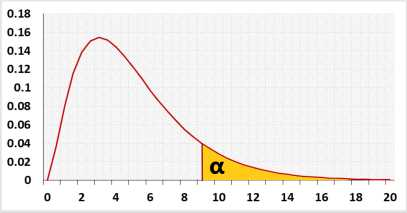# Chi-squared sample size calculatorPower analysis for the entire model

## Information

Calculate the sample size to gain the required test power and draw a power analysis chart. Use this calculator for one of the following tests:
Goodness of fit test calculator
Degrees of freedom - the number of categories minus one.
Sample size - the total number of observations across the categories.
Chi-Squared test For variance calculator
Degrees of freedom - the total number of observations minus one.
Sample size - the total number of observations.

### χ² Distribution## R Code

The following R code should produce the same results